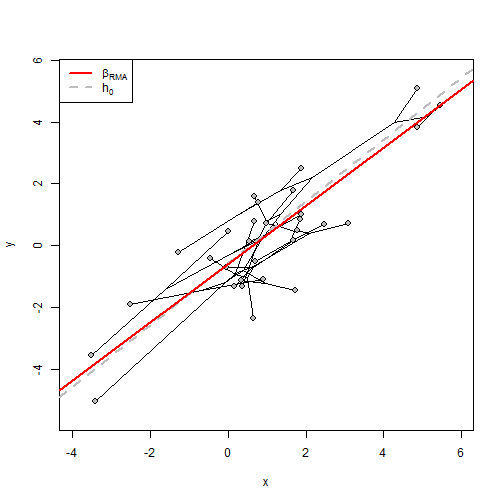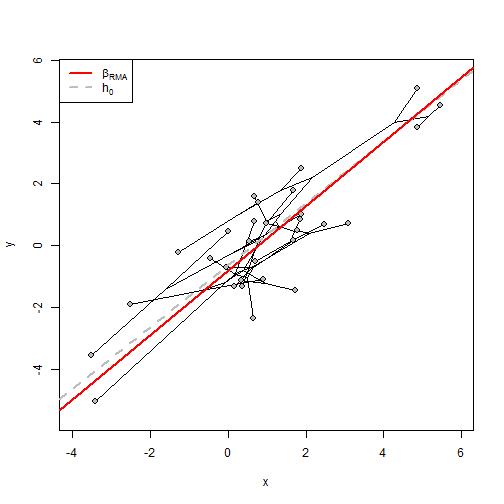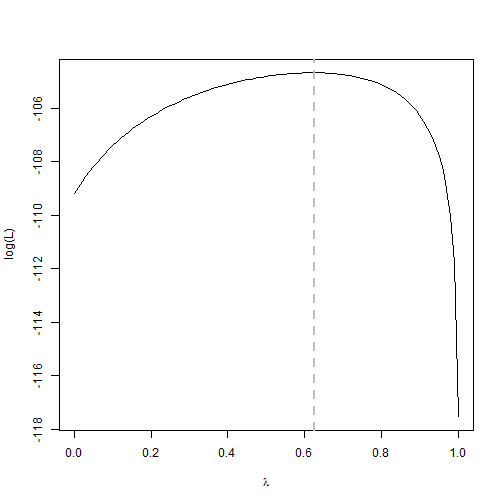## Saturday, February 25, 2017

### Testing hypotheses about Pagel's λ in `phyl.RMA`

Yesterday I received the following question by email:

“I submitted a paper for publication using your phylogenetic RMA code [`phyl.RMA(x, y, tree, method="lambda")`] and received a reviewer comment that they would like me to test whether my λ values are significantly different from zero or not. I am no R guru and have scoured the internet R forums looking for help on this topic to no avail. Do you have suggestions how to modify the code to test if λ is significantly different from 0 or not?”

This turns out to be quite straightforward now. There is no `logLik` S3 method for the `"phyl.RMA"` object class; however the likelihood is printed & it is straightforward to pull it out. The following example illustrates how we can go about doing this.

``````library(phytools)
``````

Here's our data & tree:

``````tree
``````
``````##
## Phylogenetic tree with 30 tips and 29 internal nodes.
##
## Tip labels:
##  t20, t21, t18, t19, t22, t23, ...
##
## Rooted; includes branch lengths.
``````
``````x
``````
``````##         t20         t21         t18         t19         t22         t23
##  1.74081803  0.33676433 -2.50478160  0.89857946  1.76875257  3.08699370
##         t11          t6          t3         t14         t15         t12
##  0.15106911  0.66324229 -3.41883563 -0.04319447  1.68108703  0.64884875
##          t4         t13         t27         t28         t10         t16
## -0.44859035  4.86755607  5.47266102  4.87278387  1.89486242  0.77032544
##         t17          t7          t8          t2          t1         t29
## -1.28424131  0.35679219  0.54643104  1.66794272  0.67532856  0.69751983
##         t30         t26          t9          t5         t24         t25
##  2.47659363  1.85573177  0.99397157  1.88833299 -3.51120262  0.00644626
``````
``````y
``````
``````##        t20        t21        t18        t19        t22        t23
## -1.4604984 -1.1234784 -1.8955693 -1.1046771  0.5031821  0.7232508
##        t11         t6         t3        t14        t15        t12
## -1.3182090  0.7771434 -5.0391041 -0.7060600  0.1528692 -2.3452736
##         t4        t13        t27        t28        t10        t16
## -0.4259814  5.0943036  4.5370231  3.8153191  2.4929304  1.4140231
##        t17         t7         t8         t2         t1        t29
## -0.2126191 -1.3148316  0.1247963  1.7954273  1.6049011 -0.5283464
##        t30        t26         t9         t5        t24        t25
##  0.6998745  0.8378205  0.7286870  1.0226437 -3.5451315  0.4713685
``````

Now let's fit our phylogenetic RMA:

``````## ML value of lambda
fit.ml<-phyl.RMA(x,y,tree,method="lambda")
plot(fit.ml)
````````````fit.ml
``````
``````##
## Coefficients:
## (Intercept)           x
##  -0.5950063   0.9436907
##
## VCV matrix:
##          x        y
## x 1.606109 1.267578
## y 1.267578 1.430324
##
## Model for the covariance structure of the error is "lambda"
##
## Estimates (or set values):
##       lambda       log(L)
##    0.6244482 -104.6795675
##
## Hypothesis test based on Clarke (1980; Biometrika):
##        r2         T        df         P
##  0.699423  0.559378 22.745176  0.581371
##
## Note that the null hypothesis test is h0 = 1
``````
``````## fixed lambda=0
fit.lambda0<-phyl.RMA(x,y,tree,method="lambda",
lambda=0,fixed=TRUE)
plot(fit.lambda0)
````````````fit.lambda0
``````
``````##
## Coefficients:
## (Intercept)           x
##  -0.8051448   1.0389307
##
## VCV matrix:
##          x        y
## x 1.300902 1.157591
## y 1.157591 1.404163
##
## Model for the covariance structure of the error is "lambda"
##
## Estimates (or set values):
##    lambda    log(L)
##    0.0000 -109.1883
##
## Hypothesis test based on Clarke (1980; Biometrika):
##        r2         T        df         P
##  0.733581  0.391534 22.485945  0.699089
##
## Note that the null hypothesis test is h0 = 1
``````

We can see that the ML(λ) model has a higher log-likelihood (it must have an equal or better likelihood since λ=0 is a special case); however this comparison does not tell us which model to prefer.

One option for picking the better model is through the use of a likelihood-ratio test. This is conducted as follows:

``````LR<-2*(fit.ml\$logL-fit.lambda0\$logL)
P.chisq<-pchisq(LR,df=1,lower.tail=FALSE)
P.chisq
``````
``````##  0.002674072
``````

This suggests that our more parameter-rich model fits significantly better than our simpler λ=0 model.

We can also similarly compare our ML(λ) model to a simpler Brownian model (equivalent to fixing λ=1).

``````fit.BM<-phyl.RMA(x,y,tree)
fit.BM
``````
``````##
## Coefficients:
## (Intercept)           x
##  -0.5049189   0.8606773
##
## VCV matrix:
##          x        y
## x 7.754927 5.955602
## y 5.955602 5.744582
##
## Model for the covariance structure of the error is "BM"
##
## Estimates (or set values):
##    lambda    log(L)
##    1.0000 -117.5431
##
## Hypothesis test based on Clarke (1980; Biometrika):
##        r2         T        df         P
##  0.796187  1.758561 22.027273  0.092539
##
## Note that the null hypothesis test is h0 = 1
``````
``````LR.bm<-2*(fit.ml\$logL-fit.BM\$logL)
P.bm<-pchisq(LR.bm,df=1,lower.tail=FALSE)
P.bm
``````
``````##  3.932632e-07
``````

This shows that even though our ML value of λ is closer to 1 than 0, the former is much more strongly rejected than the latter. This can be seen even more clearly if we plot our likelihood surface for λ:

``````lambda<-seq(0,1,by=0.01)
logL<-sapply(lambda,function(l,x,y,tree) phyl.RMA(x,y,tree,method="lambda",
lambda=l,fixed=TRUE)\$logL,x=x,y=y,tree=tree)
plot(lambda,logL,type="l",ylab="log(L)",xlab=expression(lambda))
abline(v=fit.ml\$lambda,lty="dashed",col="grey",lwd=2)
``````That's it.

BTW, the data & tree for this example were simulated as follows:

``````library(phytools)
tree<-pbtree(n=30)
xy<-fastBM(phytools:::lambdaTree(tree,0.6))
x<-xy+fastBM(phytools:::lambdaTree(tree,0.6),sig2=0.4)
y<-xy+fastBM(phytools:::lambdaTree(tree,0.6),sig2=0.4)
``````